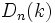# Diagonal subgroup is self-centralizing in general linear group

This article gives the statement, and possibly proof, of a particular subgroup of kind of subgroup in a group being self-centralizing. In other words, the centralizer of the subgroup in the group is contained in the subgroup
View other similar statements

## Statement

Suppose$k$ is a field with more than two elements. Then, consider the general linear group$Gl_n(k)$ of invertible$n \times n$ matrices over$k$. The subgroup$D_n(k)$ of invertible diagonal$n \times n$ matrices is self-centralizing.

(If$k$ has only two elements, the diagonal subgroup is trivial, and so clearly is not self-centralizing for$n \ge 2$).

## Proof

Given: A field$k$ with more than two elements, the group$GL_n(k)$ of invertible$n \times n$ matrices, the subgroup$D_n(k)$ of invertible diagonal matrices.

To prove:$D_n(k)$ is self-centralizing in$GL_n(k)$.

Proof: Suppose$A \in GL_n(k)$ has the property that$A$ commutes with every element of$D_n(k)$. We want to show that$A \in D_n(k)$.

Suppose not. Then, there exists a nonzero off-diagonal entry of$A$, say the$(ij)^{th}$ entry. Consider a diagonal matrix$B$ with different entries in the$(ii)$ and$(jj)$ places (for this, we need the field to have more than two elements). Then,$BA$ and$AB$ have different values in the$(ij)^{th}$ position, hence$AB \ne BA$. Hence,$A$ does not commute with every element of$D_n(k)$, leading to the required contradiction.